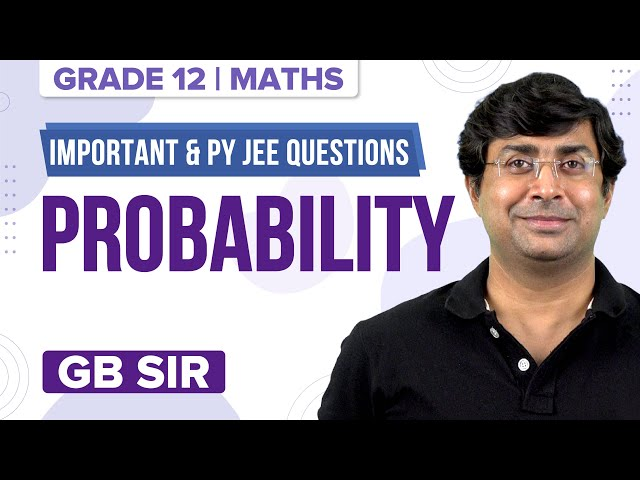Checkout JEE MAINS 2022 Question Paper Analysis : Checkout JEE MAINS 2022 Question Paper Analysis :

# How to Find Probability of an Event

Probability of an event is a number (always positive) which represents the chances of occurrence of an event. The probability range of occurrence of an event is between zero and one.  The probability of an impossible event is zero, whereas the probability of a sure event is one. Probability, as the name, deals with uncertainty. Probability problems are difficult to deal with, but it becomes easy with a little help from the subject experts. In this article, we will take a step-by-step look at what probability is and how to find the probability of an event.

Related Articles:

## What is Probability?

Probability is the likelihood of one or more events occurring. It represents the possibility of getting a certain outcome. Probability can also be described as the probability of an event occurring divided by the number of expected outcomes of the event.

## How to Calculate the Probability Step by Step

You can use the following steps to calculate the probability of an event:

Step 1: Identify an event with one result.

Step 2: Identify the total number of results or outcomes and favourable outcomes that can occur.

Step 3: Divide the number of favourable outcomes by the total number of possible outcomes.

### Formula

The most common formula used to determine the likelihood of an event is given below:

Probability of an event =

$$\begin{array}{l}\frac{Number \ of \ favourable \ outcomes}{Total \ number \ of \ outcomes}\end{array}$$

### Solved Example

Question 1:

Two dice are thrown. Find the probability of getting the sum of numbers on the dice is a perfect square.

Solution:

Total number of outcomes when two dice are thrown = 62 = 36

i.e., n(S) = 36

Let E be the event of getting the sum of numbers on the dice is a perfect square.

n(E) = Number of favourable outcomes to E = 7

since E = {(2, 2), (1, 3), (3, 1), (3, 6), (6, 3), (4, 5), (5, 4)}

P(E) = n(E)/n(S) = 7/36

Hence, the required probability is 7/36.

Question 2:

Suppose 20 balls are drawn randomly without replacement from a box containing 50 red balls and 50 black balls. What is the probability that the 10th ball is red, given that the 18th and 19th balls are red?

Solution:
Let Yi be the colour of the ith ball drawn.
Then (Y1, Y2,…., Y20) represents a random sample of size 20 without replacement from the population of 100 red and black balls.

Now,
P(Y10 = red | Y18 = red and Y19 = red) = [P(Y10 = red and Y18 = red and Y19 = red)]/[P(Y18 = red and Y19 = red]

This conditional probability is determined by the joint distribution of Y10, Y18, and Y19, which is the same as the joint distribution of Y3, Y2 and Y1, by the symmetry of sampling without replacement.
So the required probability is the same as
P(Y3 = red | Y2 = red and Y1 = red) = 48/98

### Practice Problems

1. What is the probability of a randomly chosen two-digit number being divisible by 3?

2. In a box of 10 electric bulbs, two are defective. Two bulbs are selected at random, one after the other from the box. The first bulb after selection is being put back in the box before making the second selection. What is the probability that both the bulbs are without defect?

## Probability – Important Topics## Probability – Important Questions### What are the outcomes of tossing a coin?

When a coin is tossed, the possible outcomes are head (H) and tail (T).

### What do you mean by an impossible event?

If the probability of occurrence of an event is 0, then that event is called an impossible event.

### What do you mean by a sure event?

If the probability of occurrence of an event is 1, it is called a sure event.